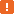# aqDateTime.Now Method### Description

Use the `aqDateTime.Now` method to get the current date and time. To get only the current date or only the current time, use the `Today` and `Time` methods respectively.

### Declaration

aqDateTime.Now()

 Result Date/Time

### Applies To

The method is applied to the following object:

### Result Value

A Date/Time value that holds the current date and time.In JavaScript, JScript, Python or DelphiScript, if you try to output the date and time returned by the `Now` method as a string, conversion takes place and the date and time are output in the native format for the selected language (`day/month/date/time/year` in JavaScript, JScript and DelphiScript, `year/month/day/time` in Python). For example, this occurs if you use the following code to post the current date and time to the test log: JavaScript, JScript function Test() {  Log.Message("Now:" + aqDateTime.Now()); } Python ``````def Test(): Log.Message("Now:" + str(aqDateTime.Now()))`````` VBScript Sub Test  Log.Message("Now:" & aqDateTime.Now())end sub DelphiScript function Test;begin  Log.Message('Now:' + aqDateTime.Now);end; C++Script, C#Script function Test() {  Log["Message"]("Now:" + aqDateTime["Now"]()); } To avoid such a conversion, you need to explicitly specify the format in which time should be displayed. For example, you can use the following code: JavaScript, JScript function Test() {  Log.Message("Now:" + aqConvert.DateTimeToFormatStr(aqDateTime.Now(), "%m/%d/%y %H:%M:%S")); } Python ``````def Test2(): Log.Message("Now:" + aqConvert.DateTimeToFormatStr(aqDateTime.Now(), "%m/%d/%y %H:%M:%S"))`````` VBScript Sub Test  Log.Message("Now:" & aqConvert.DateTimeToFormatStr(aqDateTime.Now(), "%m/%d/%y %H:%M:%S"))end sub DelphiScript function Test;begin  Log.Message('Now:' + aqConvert.DateTimeToFormatStr(aqDateTime.Now(), '%m/%d/%y %H:%M:%S'));end; C++Script, C#Script function Test() {  Log["Message"]("Now:" + aqConvert["DateTimeToFormatStr"](aqDateTime["Now"](), "%m/%d/%y %H:%M:%S")); }

### Example

The code below demonstrates how you can compare different date and time values.

JavaScript, JScript

function ComparingDates()
{
var currentDateAndTime = aqDateTime.Now();
var currentTime = aqDateTime.Time();
var currentDate = aqDateTime.Today();

Log.Message(currentDateAndTime);
Log.Message(currentTime);
Log.Message(currentDate);

Log.Message(aqDateTime.Compare(currentDateAndTime, currentTime));
// 1, because currentDateAndTime is later then currentTime

Log.Message(aqDateTime.Compare(currentDateAndTime, currentDate));
// 1, because currentDateAndTime is later then currentDate

Log.Message(aqDateTime.Compare(currentTime, currentDate));
// -1, because currentTime is earlier then currentDate
}

Python

``````def ComparingDates():
currentDateAndTime = aqDateTime.Now()
currentTime = aqDateTime.Time()
currentDate = aqDateTime.Today()
Log.Message(currentDateAndTime)
Log.Message(currentTime)
Log.Message(currentDate)
Log.Message(aqDateTime.Compare(currentDateAndTime, currentTime))
# 1, because currentDateAndTime is later then currentTime
Log.Message(aqDateTime.Compare(currentDateAndTime, currentDate));
# 1, because currentDateAndTime is later then currentDate
Log.Message(aqDateTime.Compare(currentTime, currentDate));
# -1, because currentTime is earlier then currentDate``````

VBScript

Sub ComparingDates
Dim currentDateAndTime, currentTime, currentDate

currentDateAndTime = aqDateTime.Now
currentTime = aqDateTime.Time
currentDate = aqDateTime.Today

Log.Message(currentDateAndTime)
Log.Message(currentTime)
Log.Message(currentDate)

Log.Message(aqDateTime.Compare(currentDateAndTime, currentTime))
' 1, because currentDateAndTime is later then currentTime

Log.Message(aqDateTime.Compare(currentDateAndTime, currentDate))
' 1, because currentDateAndTime is later then currentDate

Log.Message(aqDateTime.Compare(currentTime, currentDate))
' -1, because currentTime is earlier then currentDate
End Sub

DelphiScript

procedure ComparingDates;
var currentDateAndTime, currentTime, currentDate;
begin
currentDateAndTime := aqDateTime.Now;
currentTime := aqDateTime.Time;
currentDate := aqDateTime.Today;

Log.Message(currentDateAndTime);
Log.Message(currentTime);
Log.Message(currentDate);

Log.Message(aqDateTime.Compare(currentDateAndTime, currentTime));
// 1, because currentDateAndTime is later then currentTime

Log.Message(aqDateTime.Compare(currentDateAndTime, currentDate));
// 1, because currentDateAndTime is later then currentDate

Log.Message(aqDateTime.Compare(currentTime, currentDate));
// -1, because currentTime is earlier then currentDate
end;

C++Script, C#Script

function ComparingDates()
{
var currentDateAndTime = aqDateTime["Now"]();
var currentTime = aqDateTime["Time"]();
var currentDate = aqDateTime["Today"]();

Log["Message"](currentDateAndTime);
Log["Message"](currentTime);
Log["Message"](currentDate);

Log["Message"](aqDateTime["Compare"](currentDateAndTime, currentTime));
// 1, because currentDateAndTime is later then currentTime

Log["Message"](aqDateTime["Compare"](currentDateAndTime, currentDate));
// 1, because currentDateAndTime is later then currentDate

Log["Message"](aqDateTime["Compare"](currentTime, currentDate));
// -1, because currentTime is earlier then currentDate
}